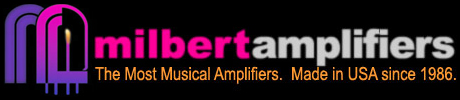# Milbert Amplifiers

The Most Musical Amplifiers. Made in USA since 1986.

## ArticlesBlogShowssoundQuality >

### How Loud is 5 Watts

Shout really loud -- that's how loud 5 watts is. "Heaphone level" level corresponds to about 20 mW, about the power available from a small signal tube amp. Thus a 5 watt amp is 5 / .002 = 2,500 times louder than the desired 20 mW "headphone level". Human perception, like most natural response, abides a logarithmic function.

"Last week, I played a 35W amp on full blast (but not clipping) through a Marshall 4x12" cabinet loaded with Celestion Vintage 30's, which have a sensitivity of 100dB. I took out a sound pressure level meter and put it 10 feet away from the speakers. The 35W amp produced a clean power level of 117dB, which is only 3dB quieter than an airplane landing." »

Human "loudness" perception, relative to 50 watts:

500 W - tenfold increase in power "sounds about" twice as loud.
100 W - twofold increase in power "sounds about" 25% louder.
50 W - unity.
15 W - threefold decrease in power "sounds about" 2/3rds as loud.
5 W - tenfold decrease in power (1 order of magnitude) "sounds about" 1/2 as loud.
0.5 W - hundredfold decrease in power (2 orders of magnitude) "sounds about" 1/4th as loud.
0.005 W (50 mW) - thousandfold decrease in power (3 orders of magnitude) "sounds about" 1/8th as loud.

A 5 watt amp is *a thousand times too powerful* and "sounds about" eight times too loud, compared to the target "headphone level" of 20 mW.

X% louder = 2^log10(P2/P1) * 100%

40 watts is 94% as loud as 50 watts.
30 watts is 86% as loud as 50 watts.

25 watts is 81% as loud as 50 watts.
22 watts is 78% as loud as 50 watts.

20 watts is 76% as loud as 50 watts.
18 watts is 74% as loud as 50 watts.

15 watts is 70% as loud as 50 watts.
12 watts is 65% as loud as 50 watts.

10 watts is 62% as loud as 50 watts.
9 watts is 60% as loud as 50 watts.

8 watts is 56% as loud as 50 watts.
7 watts is 55% as loud as 50 watts.

6 watts is 53% as loud as 50 watts.
5 watts is 50% as loud as 50 watts.

4 watts is 47% as loud as 50 watts.
3 watts is 43% as loud as 50 watts.

2 watts is 38% as loud as 50 watts.
1 watt is 31% as loud as 50 watts.

3/4 watt is 28% as loud as 50 watts.
1/2 watt is 25% as loud as 50 watts.

1/4 watt is 20% as loud as 50 watts.
1/10 watt is 15% as loud as 50 watts.

50mW is 13% as loud as 50 watts
20mW is 10% as loud as 50 watts.

10mW is 8% as loud as 50 watts.
5mW is 6% as loud as 50 watts.

1mW is 4% as loud as 50 watts.
0.5mW is 3% as loud as 50 watts.

0.1mW is 2% as loud as 50 watts.
50uW is 1.6% as loud as 50 watts.

10uW is 1% as loud as 50 watts.

Material herein added and updated constantly; presented for inspirational and educational purposes per Fair Use.